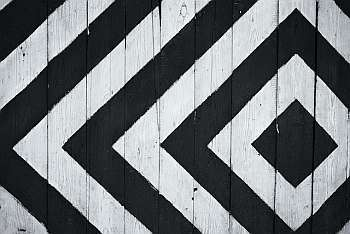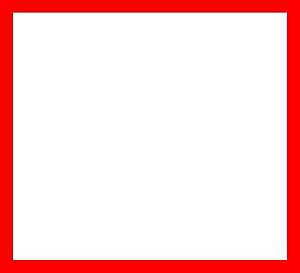# Area of a Square

Instructions: Use this calculator to find the area of square, with a given side s. Please provide the side in the form box below.The side $$s$$ of the square (Ex: 2, or 3/2, etc.)

This calculator is computes the area of a square, for a side that you provide. The provided side of the square can be any valid numeric expression. For example it could any number like '3' or '6.56', or you could use expressions like '1/3', 'sqrt(3)', etc.

Once you have provided a valid expression, all you have to do is to click on "Calculate", and all the steps will be shown.

The process is quite simple and it just involves squaring the value of the side provided.## How do compute the area of a square?

The actual calculation is very simple, and it consists of simply multiplying the side of the square by itself. So, if the side of the square is $$a$$, then the formula for the area of a square is

$\text{Area} = a^2$

## What are the steps for computing the area of a square

• Step 1: Identify the side that is being provided, and call that side 'a'
• Step 2: Once you know the side 'a', the area is computed a * a = a²
• Step 3: If needed, identify the units of 'a' (if any) and give units to the area

## Why would calculate the area of a square?

There are countless applications that involve the calculation of areas of squares. For example, you may be interested in calculating the square footage of a square plot of land, for which purpose you would use the formula for the area of a square.

The areas of squares and rectangles is the basis for the definition of non-straight surfaces, in the context for example of integral calculus.### Example: Calculating the area of a square

Calculate the area of square of side a = 4.5.

Solution: We first identify the side of the square we need to use. In this case it is clear that a = 4.5. Second, the formula for the area is:

$Area = a^2$

Then, by plugging a = 4.5 into the formula:

$Area = a^2 = 4.5^2 = 20.25$

### Example: Another area calculation

Calculate the area of square with a diagonal d = 5.

Solution: In order to use the formula we know, we first identify the side of the square we need to use. But instead of the side, we have been provided with the diagonal.

By Pythagorean theorem, we know that $$d = a \sqrt{2}$$, where d is the diagonal and a is the side. So then, we can solve for the side:

$d = a \sqrt{2} \Rightarrow a = \displaystyle\frac{d}{\sqrt{2}}$

In this case, we have d = 5:

$a = \displaystyle\frac{d}{\sqrt{2}} = \displaystyle\frac{5}{\sqrt{2}}$

Then, by plugging $$a = \displaystyle\frac{5}{\sqrt{2}}$$ into the formula:

$Area = a^2 = \left(\frac{5}{\sqrt{2}}\right)^2 = \frac{25}{2} = 12.5$

### Example: Area of a square with units

Calculate the area of square with a side of a = 4 cm

Solution: We first identify the side of the square we need to use, which is a = 4 cm in this case. Observe that a comes with a type of unit.

$Area = a^2$

Then, by plugging a = 4 cm into the formula:

$Area = a^2 = 4^2 cm^2 = 16 cm^2$

## Other useful area calculators

Other geometric shapes can also be required. You can calculate the area of a rectangle, for example, using a very simple formula. Slightly more complicated is the case of the area of a rhombus, but still follows the same rationale, which is also similar to that is used for the calculation of the area of a triangle.

In a different category, because of the involvement of the constant $$\pi$$, you can use our calculators for the area of circle and the area of an ellipse, which are remarkably similar.